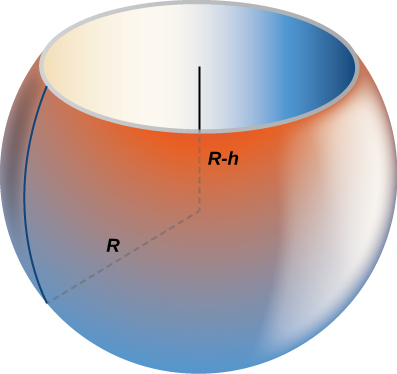## Problem Set: Determining Volumes by Slicing

1. Derive the formula for the volume of a sphere using the slicing method.

2. Use the slicing method to derive the formula for the volume of a cone.

3. Use the slicing method to derive the formula for the volume of a tetrahedron with side length $a.$

4. Use the disk method to derive the formula for the volume of a trapezoidal cylinder.

5. Explain when you would use the disk method versus the washer method. When are they interchangeable?

For the following exercises (6-10), draw a typical slice and find the volume using the slicing method for the given volume.

6. A pyramid with height 6 units and square base of side 2 units, as pictured here.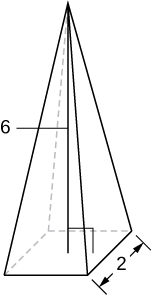7. A pyramid with height 4 units and a rectangular base with length 2 units and width 3 units, as pictured here.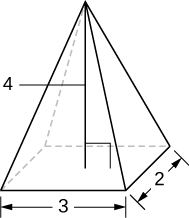8. A tetrahedron with a base side of 4 units, as seen here.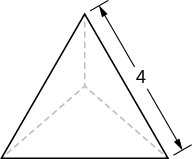9. A pyramid with height 5 units, and an isosceles triangular base with lengths of 6 units and 8 units, as seen here.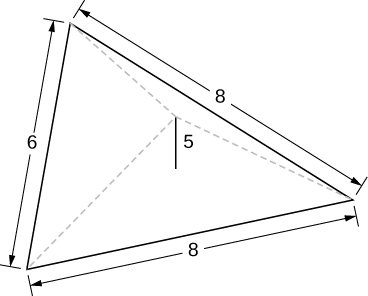10. A cone of radius $r$ and height $h$ has a smaller cone of radius $r\text{/}2$ and height $h\text{/}2$ removed from the top, as seen here. The resulting solid is called a frustum.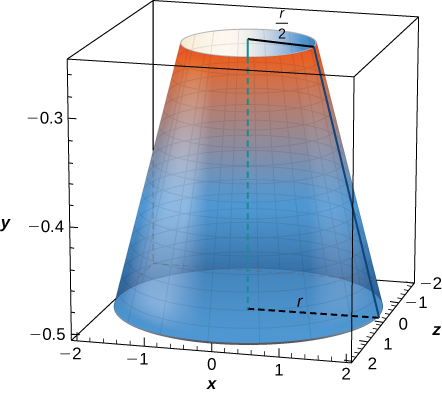For the following exercises (11-16), draw an outline of the solid and find the volume using the slicing method.

11. The base is a circle of radius $a.$ The slices perpendicular to the base are squares.

12. The base is a triangle with vertices $(0,0),(1,0),$ and $(0,1).$ Slices perpendicular to the xy-plane are semicircles.

13. The base is the region under the parabola $y=1-{x}^{2}$ in the first quadrant. Slices perpendicular to the xy-plane are squares.

14. The base is the region under the parabola $y=1-{x}^{2}$ and above the $x\text{-axis}\text{.}$ Slices perpendicular to the $y\text{-axis}$ are squares.

15. The base is the region enclosed by $y={x}^{2}$ and $y=9.$ Slices perpendicular to the $x$-axis are right isosceles triangles.

16. The base is the area between $y=x$ and $y={x}^{2}.$ Slices perpendicular to the $x$-axis are semicircles.

For the following exercises (17-24), draw the region bounded by the curves. Then, use the disk method to find the volume when the region is rotated around the $x$-axis.

17. $x+y=8,x=0,\text{ and }y=0$

18. $y=2{x}^{2},x=0,x=4,\text{ and }y=0$

19. $y={e}^{x}+1,x=0,x=1,\text{ and }y=0$

20. $y={x}^{4},x=0,\text{ and }y=1$

21. $y=\sqrt{x},x=0,x=4,\text{ and }y=0$

22. $y= \sin x,y= \cos x,\text{ and }x=0$

23. $y=\frac{1}{x},x=2,\text{ and }y=3$

24. ${x}^{2}-{y}^{2}=9\text{ and }x+y=9,y=0\text{ and }x=0$

For the following exercises (25-32), draw the region bounded by the curves. Then, find the volume when the region is rotated around the $y$-axis.

25. $y=4-\frac{1}{2}x,x=0,\text{ and }y=0$

26. $y=2{x}^{3},x=0,x=1,\text{ and }y=0$

27. $y=3{x}^{2},x=0,\text{ and }y=3$

28. $y=\sqrt{4-{x}^{2}},y=0,\text{ and }x=0$

29. $y=\frac{1}{\sqrt{x+1}},x=0,\text{ and }x=3$

30. $x= \sec (y)\text{ and }y=\frac{\pi }{4},y=0\text{ and }x=0$

31. $y=\frac{1}{x+1},x=0,\text{ and }x=2$

32. $y=4-x,y=x,\text{ and }x=0$

For the following exercises (33-40), draw the region bounded by the curves. Then, find the volume when the region is rotated around the $x$-axis.

33. $y=x+2,y=x+6,x=0,\text{ and }x=5$

34. $y={x}^{2}\text{ and }y=x+2$

35. ${x}^{2}={y}^{3}\text{ and }{x}^{3}={y}^{2}$

36. $y=4-{x}^{2}\text{ and }y=2-x$

37. [T] $y= \cos x,y={e}^{\text{−}x},x=0,\text{ and }x=1.2927$

38. $y=\sqrt{x}\text{ and }y={x}^{2}$

39. $y= \sin x\text{,}y=5 \sin x,x=0\text{ and }x=\pi$

40. $y=\sqrt{1+{x}^{2}}\text{ and }y=\sqrt{4-{x}^{2}}$

For the following exercises (41-45), draw the region bounded by the curves. Then, use the washer method to find the volume when the region is revolved around the $y$-axis.

41. $y=\sqrt{x},x=4,\text{ and }y=0$

42. $y=x+2,y=2x-1,\text{ and }x=0$

43. $y=\sqrt{x}\text{ and }y={x}^{3}$

44. $x={e}^{2y},x={y}^{2},y=0,\text{ and }y=\text{ln}(2)$

45. $x=\sqrt{9-{y}^{2}},x={e}^{\text{−}y},y=0,\text{ and }y=3$

46. Yogurt containers can be shaped like frustums. Rotate the line $y=\frac{1}{m}x$ around the $y$-axis to find the volume between $y=a\text{ and }y=b.$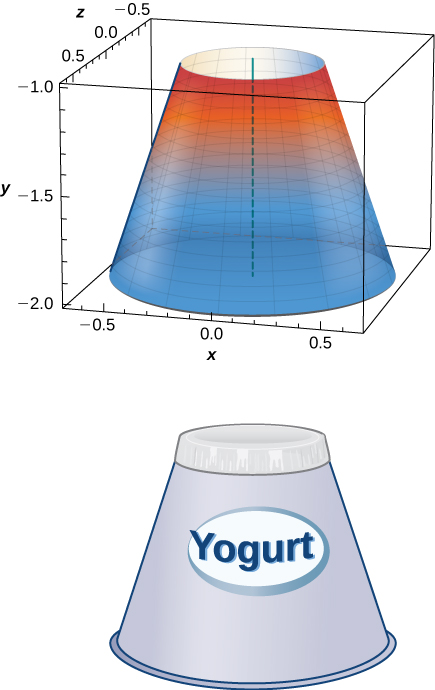47. Rotate the ellipse $({x}^{2}\text{/}{a}^{2})+({y}^{2}\text{/}{b}^{2})=1$ around the $x$-axis to approximate the volume of a football, as seen here.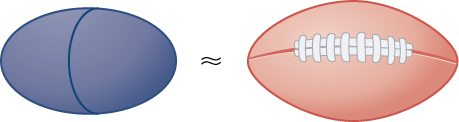48. Rotate the ellipse $({x}^{2}\text{/}{a}^{2})+({y}^{2}\text{/}{b}^{2})=1$ around the $y$-axis to approximate the volume of a football.

49. A better approximation of the volume of a football is given by the solid that comes from rotating $y= \sin x$ around the $x$-axis from $x=0$ to $x=\pi .$ What is the volume of this football approximation, as seen here?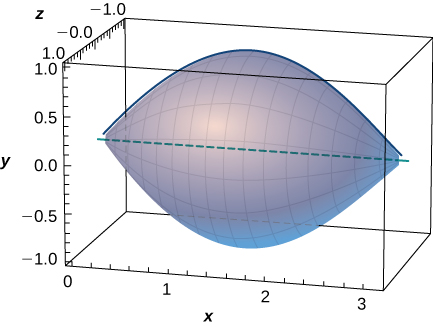50. What is the volume of the Bundt cake that comes from rotating $y= \sin x$ around the $y$-axis from $x=0$ to $x=\pi ?$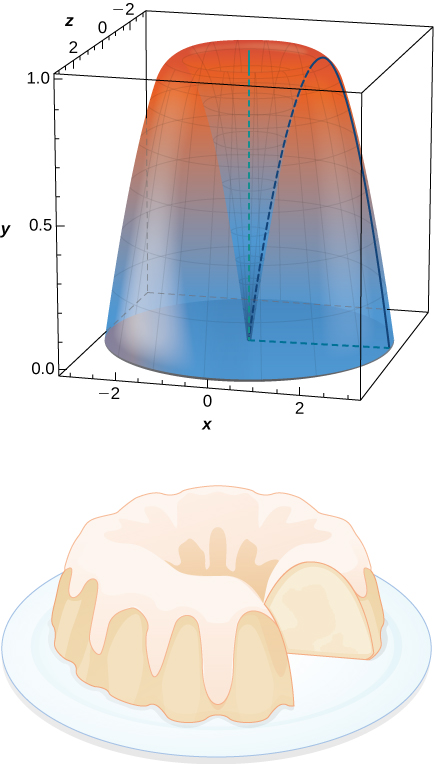For the following exercises (51-56), find the volume of the solid described.

51. The base is the region between $y=x$ and $y={x}^{2}.$ Slices perpendicular to the $x$-axis are semicircles.

52. The base is the region enclosed by the generic ellipse $({x}^{2}\text{/}{a}^{2})+({y}^{2}\text{/}{b}^{2})=1.$ Slices perpendicular to the $x$-axis are semicircles.

53. Bore a hole of radius $a$ down the axis of a right cone and through the base of radius $b,$ as seen here.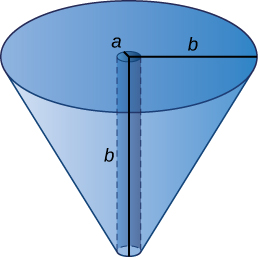54. Find the volume common to two spheres of radius $r$ with centers that are $2h$ apart, as shown here.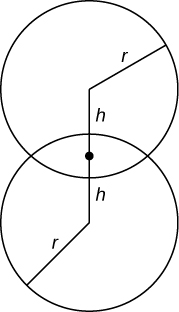55. Find the volume of a spherical cap of height $h$ and radius $r$ where $h<r,$ as seen here.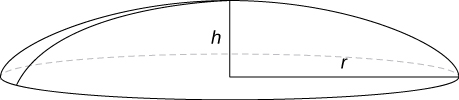56. Find the volume of a sphere of radius $R$ with a cap of height $h$ removed from the top, as seen here.